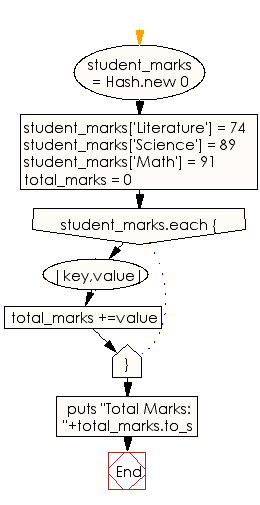﻿ Ruby Basic exercises: Retrieve the student information from a hash - w3resource# Ruby Basic Exercises: Retrieve the student information from a hash

## Ruby Basic: Exercise-31 with Solution

Write a Ruby program to retrieve the total marks where subject name and marks of a student stored in a hash.

Sample subject and marks : Literature -74, Science – 89, Math-91

Ruby Code:

``````student_marks = Hash.new 0
student_marks['Literature'] = 74
student_marks['Science'] = 89
student_marks['Math'] = 91
total_marks = 0
student_marks.each {|key,value|
total_marks +=value
}
puts "Total Marks: "+total_marks.to_s
``````

Output:

```Total Marks: 254
```

Flowchart:Ruby Code Editor: